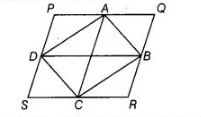# The quadrilateral formed by joining`
Question:

The quadrilateral formed by joining the mid-points of the sides of a quadrilateral $\mathrm{PQRS}$, taken in order, is a rectangle, if

(a) PQRS is a rectangle

(b) PQRS is a parallelogram

(c) diagonals of PQRS are perpendicular

(d) diagonals of $P Q R S$ are equal

Solution:

(c) Since, the quadrilateral $A B C D$ formed by joining the mid-points of quadrilateral $P Q R S$ is a rectangle.

$A C=B D[$ since, diagonals of a rectangle are equal] $\Rightarrow P Q=Q R$

Thus, quadrilateral $P Q R S$ is a rhombus.Hence, diagonals of $P Q R S$ i.e., $P R$ and $Q S$ are perpendicular.

[since, diagonals of rhombus are perpendicular to each other]